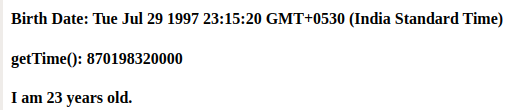Related Articles

# JavaScript Date getTime() Method

• Last Updated : 19 Oct, 2021

Below is the example of Date getTime() method.

• Example:

## JavaScript

 ``
• Output:

`845337932000`

The date.getTime() method method used to returns the number of milliseconds since 1 January 1970. when a new Date object is created it stores the date and time data when it is created. When the getTime() method is called on this date object it returns the number of milliseconds since 1 January 1970 (Unix Epoch).
Syntax:

`Date.getTime()`

Parameters: This method does not accept any parameter.
Return type: A numeric value equal to no of milliseconds since Unix Epoch.
More codes for the above method are as follows:
Program 1: Here the date of the month must lie in between 1 to 31 because no date can have month greater than 31. That is why it returns NaN i.e, Not a Number if the month in the Date object is greater than 31. Hours will not have existed when the date of the month given as 33 i.e, greater than 31.

## JavaScript

 ``

Output:

`NaN`

Program 2: Here we will calculate the user age by providing the birth date of the user.

## html

 ```<``html` `lang``=``"en"``>` `<``head``>``    ``<``meta` `charset``=``"UTF-8"``>``    ``<``meta` `name``=``"viewport"``        ``content="``width``=``device``-width,``                ``initial-scale``=``1``.0">``    ``<``meta` `http-equiv``=``"X-UA-Compatible"``        ``content``=``"ie=edge"``>``    ``<``title``>Document``` `<``body``>``    ``<``div``>``        ``<``h4``>Birth Date:``            ``<``span` `class``=``"date"``>``        ````    ````    ``<``div``>``        ``<``h4``>getTime():``            ``<``span` `class``=``"time"``>``        ````    ````    ``<``div``>``        ``<``h4``>I am``            ``<``span` `class``=``"years"``>``            ``years old.``        ````    ``````<``script``>``    ``var BD = new Date("July 29, 1997 23:15:20");``    ``var date = document.querySelector(".date");``    ``var time = document.querySelector(".time");``    ``var Today = new Date();``    ``var today = Today.getTime();``    ``var bd = BD.getTime();``    ``var year = 1000 * 60 * 60 * 24 * 365;``    ``var years = (today - bd) / year;``    ``date.innerHTML = BD;``    ``time.innerHTML = BD.getTime();``    ``var y = document.querySelector(".years");``    ``y.innerHTML = Math.round(years);``` `                   `

Output:Supported Browsers: The browsers supported by JavaScript Date getTime() method are listed below:

• Google Chrome 1 and above
• Edge 12 and above
• Firefox 1 and above
• Internet Explorer 4 and above
• Opera 3 and above
• Safari 1 and above

Hey geek! The constant emerging technologies in the world of web development always keeps the excitement for this subject through the roof. But before you tackle the big projects, we suggest you start by learning the basics. Kickstart your web development journey by learning JS concepts with our JavaScript Course. Now at it’s lowest price ever!

My Personal Notes arrow_drop_up